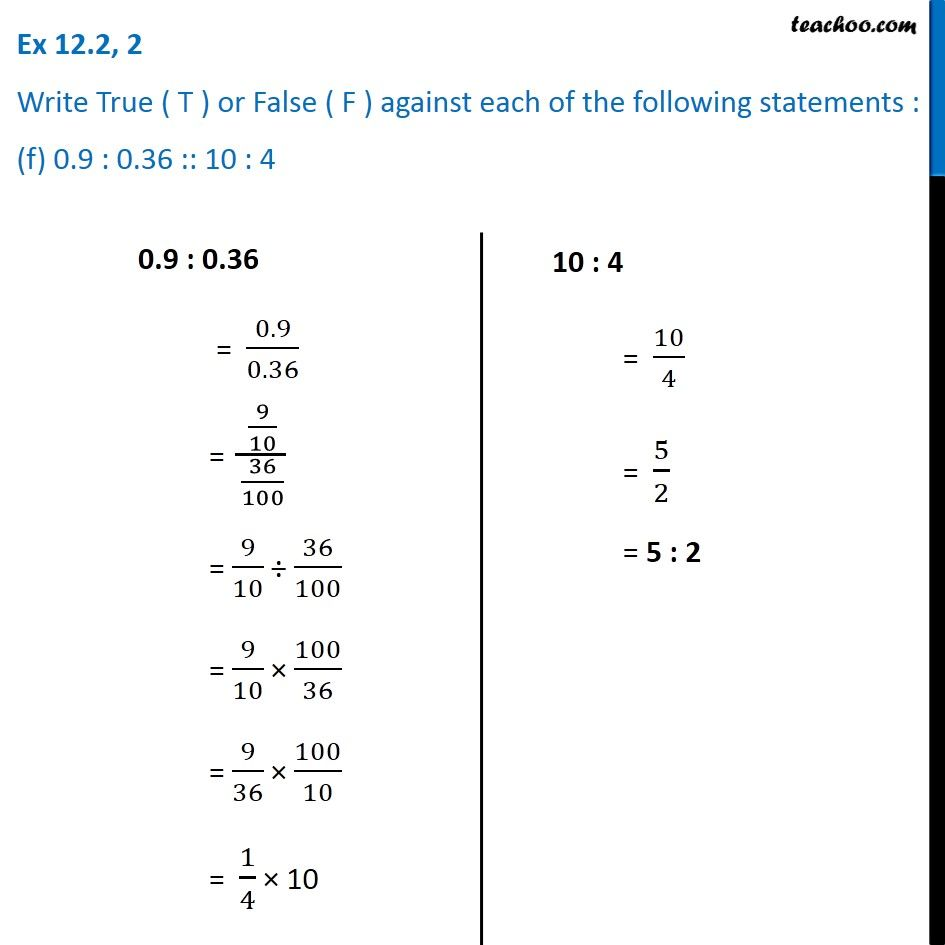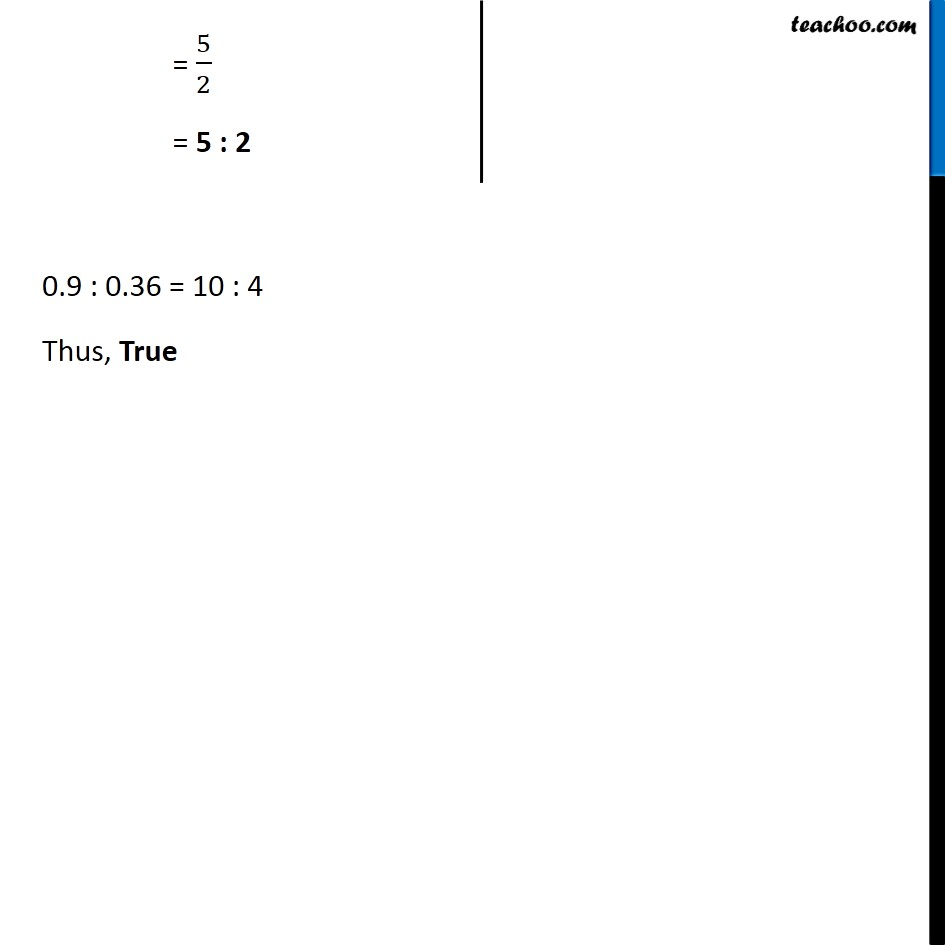Ex 12.2

Chapter 12 Class 6 Ratio and Proportion
Serial order wiseLearn in your speed, with individual attention - Teachoo Maths 1-on-1 Class

### Transcript

Ex 12.2, 2 Write True ( T ) or False ( F ) against each of the following statements : (f) 0.9 : 0.36 :: 10 : 40.9 : 0.36 = 0.9/0.36 = (9/10)/(36/100) = 9/10 ÷ 36/100 = 9/10 × 100/36 = 9/36 × 100/10 = 1/4 × 10 10 : 4 = 10/4 = 5/2 = 5 : 2 = 5/2 = 5 : 2 0.9 : 0.36 = 10 : 4 Thus, True